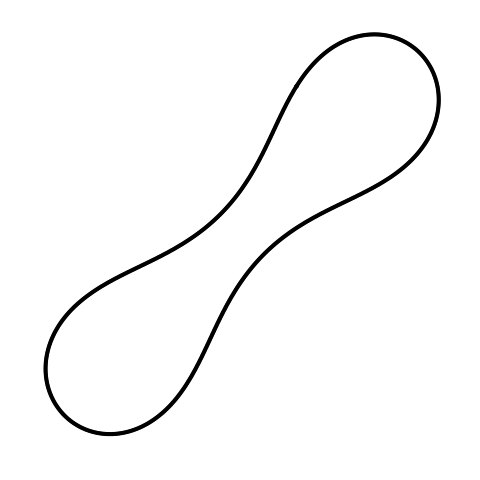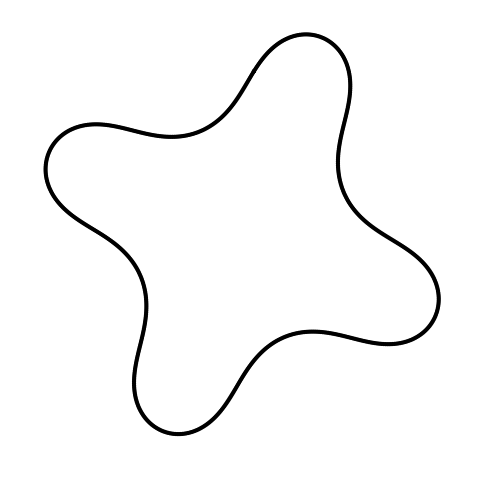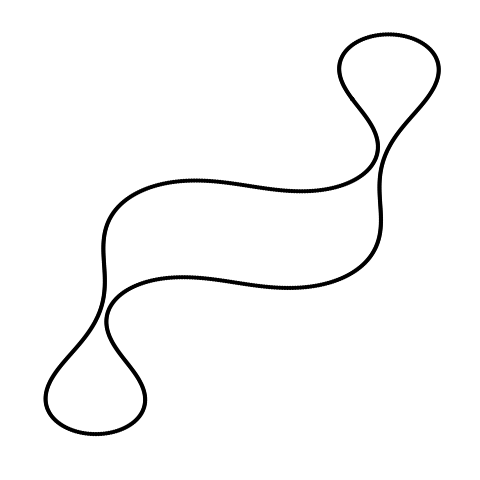Want to share your content on R-bloggers? click here if you have a blog, or here if you don't.

### Introduction

I’ve just uploaded a new package to CRAN based on a stimulus generation algorithm that I use for my experiments on vision. The FourierDescriptors package provides methods for creating, manipulating and visualizing Fourier descriptors, which are a representational scheme used to describe closed planar contours. The canonical reference from the literature is Zahn and Roskies 1972. The images most easily described using Fourier descriptors are useful as stimuli for experiments in psychology and neuroscience.

### Installation

This package has been submitted to CRAN. When it propagates through the mirrors, you can install it using a simple call to `install.packages()`:

 ```1 ``` `install.packages('FourierDescriptors')`

 ```1 ``` `R CMD INSTALL FourierDescriptors_*.tar.gz`

### Basic Usage Example

The following example showcases the central methods that currently exist in the FourierDescriptors package:

 ```1 2 3 4 5 6 7 8 ``` ```library('FourierDescriptors')   fd1 <- create.fourier.descriptor() print(fd1) plot(fd1)   fd2 <- random.fourier.descriptor(32, 2) plot(fd2)```

### Gaining Intuition about the Amplitude Spectrum

It’s useful to see the images generated by very basic amplitude spectra to see how these determine the shape of the induced contour.

 ```1 2 ``` ```library('FourierDescriptors') plot(create.fourier.descriptor(amplitude = c(0, 0, 0, 0)))``````1 ``` `plot(create.fourier.descriptor(amplitude = c(0, 1, 0, 0)))````1 ``` `plot(create.fourier.descriptor(amplitude = c(0, 0, 0, 1)))````1 ``` `plot(create.fourier.descriptor(amplitude = c(0, 1, 0, 1)))````1 2 3 4 ``` ```plot(random.fourier.descriptor(24, non.zero.frequencies = 4, generating.function = function() {runif(1)}), steps = 360 * 10)```Please note that only even-numbered frequencies can have non-zero amplitudes or the described curve will not be closed.